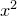# 11.57 tabulate

```tabulate [ <range> ]
( <filename> | { <expression> } | { <vector obj> } )
[ every { <expression> } ]
[ index <value> ]
[ select <expression> ]
[ sortby <expression> ]
[ using { <expression> } ]
[ with <output format> ]
```

Pyxplot’s tabulate command is similar to its plot command, but instead of plotting a series of data points onto a graph, it outputs them to data files. This can be used to produce text files containing samples of functions, to rearrange/filter the columns in data files, to change the units in which data is expressed in data files, and so forth. The following example would produce a data file called gamma.dat containing a list of values of the gamma function:

```set output 'gamma.dat'
tabulate [1:5] gamma(x)
```

Multiple functions may be tabulated into the same file, either by using the using modifier:

```tabulate [0:2*pi] sin(x):cos(x):tan(x) u 1:2:3:4
```

or by placing them in a comma-separated list, as in the plot command:

```tabulate [0:2*pi] sin(x), cos(x), tan(x)
```

In the former case, the functions are tabulated horizontally alongside one another in a series of columns. In the latter case, the functions are tabulated one after another in a series of index blocks separated by double linefeeds (see Section 3.8).

The setting samples can be used to control the number of points that are produced when tabulating functions, in the same way that it controls the plot command:

```set samples 200
```

If the ordinate axis is set to be logarithmic then the points at which functions are evaluated are spaced logarithmically, otherwise they are spaced linearly.

The select, using and every modifiers operate in the same manner in the tabulate command as in the plot command. Thus, the following example would write out the third, sixth and ninth columns of the data file input.dat, but only when the arcsine of the value in the fourth column is positive:

```set output 'filtered.dat'
tabulate 'input.dat' u 3:6:9 select (asin(\$4)>0)
```

The numerical display format used in each column of the output file is chosen automatically to preserve accuracy whilst simultaneously being as easily human readable as possible. Thus, columns which contain only integers are displayed as such, and scientific notation is only used in columns which contain very large or very small values. If desired, however, a format statement may be specified using the with format specifier. The syntax for this is similar to that expected by the string substitution operator (%; see Section 6.2.1). As an example, to tabulate the values ofto very many significant figures with some additional text, one could use:

```tabulate x**2 with format "x = %f ; x**2 = %27.20e"
```

This might produce the following output:

```x = 0.000000 ; x**2 =  0.00000000000000000000e+00
x = 0.833333 ; x**2 =  6.94444444444442421371e-01
x = 1.666667 ; x**2 =  2.77777777777778167589e+00
```

The data produced by the tabulate command can be sorted in order of any arbitrary metric by supplying an expression after the sortby modifier; where such expressions are supplied, the data is sorted in order from the smallest value of the expression to the largest.